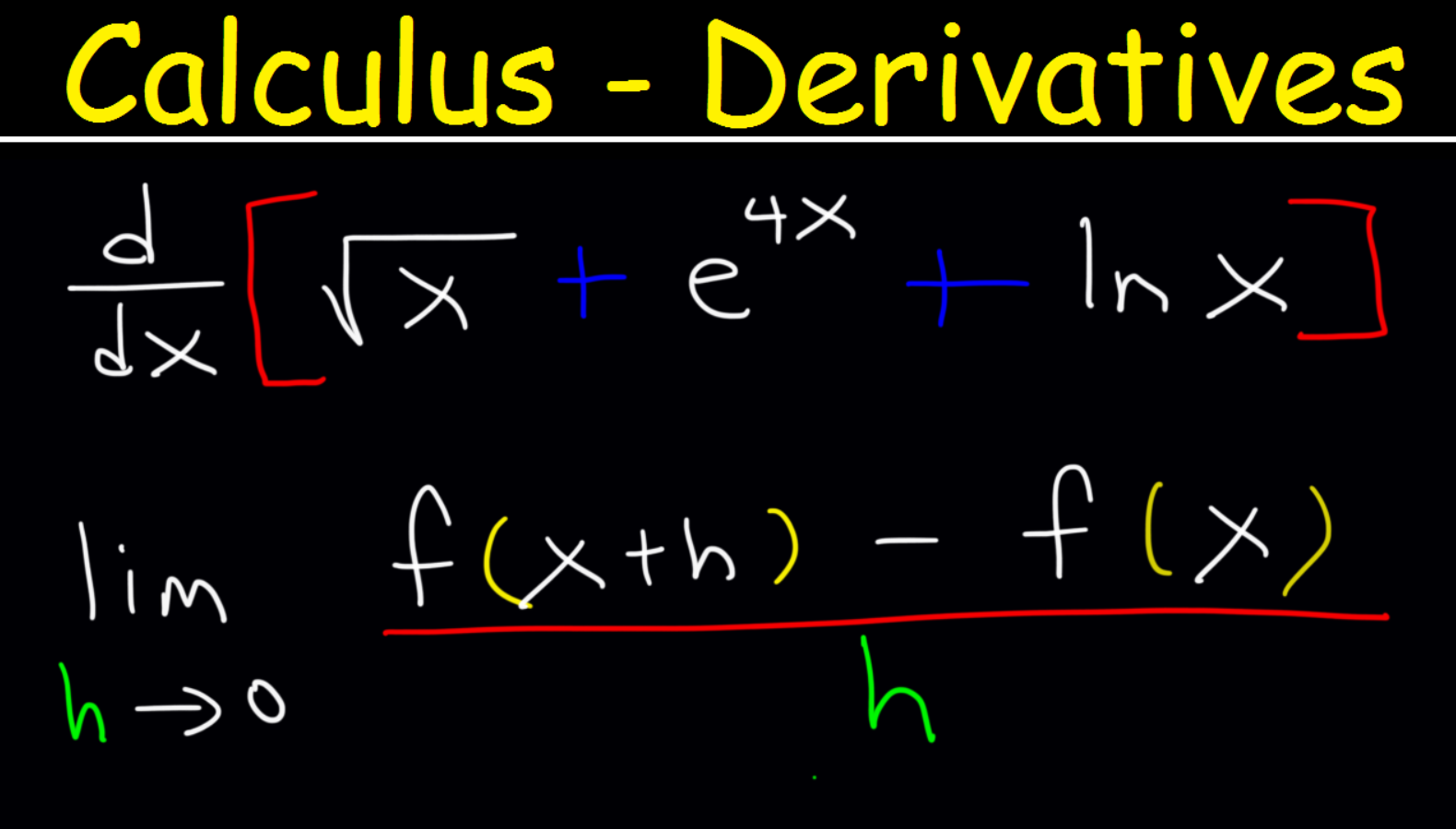Calculus 1 Derivatives - Product & Quotient Rule, Chain Rule, Exponential & Logarithmic Functions | Julio Gonzalez | Skillshare

# Calculus 1 Derivatives - Product & Quotient Rule, Chain Rule, Exponential & Logarithmic Functions

#### Julio Gonzalez

Play Speed
• 0.5x
• 1x (Normal)
• 1.25x
• 1.5x
• 2x
25 Videos (2h 25m)
• Lesson 1 The Constant Rule

1:51
• Lesson 2 The Power Rule

1:50
• Lesson 3 The Constant Multiple Rule

3:10
• Lesson 4 Derivatives of Rational Functions

3:10
• Lesson 5 Square Roots and Radicals

7:51
• Lesson 6 Derivatives of Basic Trigonometric Functions

2:22
• Lesson 7 Sum and Difference Rules

3:46
• Lesson 8 Average Rate of Change vs Instantaneous Rate of Change

7:38
• Lesson 9 Estimating The Instantaneous Rate of Change Given a Table of Values

3:22
• Lesson 12 Limits and Derivatives More Examples

2:09
• Lesson 10 Tangent Line Equation

6:19
• Lesson 11 Limit Definition of the Derivative

9:36
• Lesson 12 Limits and Derivatives More Examples

2:09
• Lesson 13 Alternative Form of the Derivative

5:50
• Lesson 14 Derivative of Exponential Functions

3:00
• Lesson 15 Derivative of Natural Log Functions

1:51
• Lesson 16 Derivatives of Logarithmic Functions

2:25
• Lesson 17 The Product Rule

6:21
• Lesson 18 The Quotient Rule

6:05
• Lesson 19 The Chain Rule

18:17
• Lesson 20 Implicit Differentiation

6:15
• Lesson 21 Inverse Trig Functions

4:03
• Lesson 22 Logarithmic Differentiation

7:26
• Video Quiz 1

15:24
• Video Quiz 2

13:19

This calculus 1 video course explains how to find the derivative of multiple functions.  This course is for college students taking their first semester of calculus or high school students taking AP calculus AB. This video contains plenty of examples and practice problems.

Here is a list of topics:

1.  The Constant Rule

2.  Power Rule and Constant Multiple Rule

3.  Derivatives of Polynomial Functions Using The Sum and Difference Rule

4.  Derivatives of Rational Functions / Fractions

5.  Differentiating Square Roots and Radicals

6.  Average Rate of Change vs Instantaneous Rate of Change

7.  Tangent Line Equations

8.  Product Rule and Quotient Rule

9.  Derivative of Composite Functions Using The Chain Rule

10.  Limit Definition of the Derivative

11.  Derivatives of Exponential Functions and Natural Logs

12.  Logarithmic Differentiation

13.  Free response video quiz31

Students

--

Projects

• --
• Beginner
• Intermediate